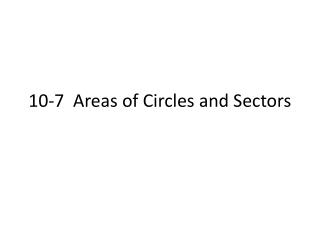DownloadDownload Presentation10-7 Areas of Circles and Sectors

# 10-7 Areas of Circles and Sectors

Télécharger la présentation## 10-7 Areas of Circles and Sectors

- - - - - - - - - - - - - - - - - - - - - - - - - - - E N D - - - - - - - - - - - - - - - - - - - - - - - - - - -
##### Presentation Transcript

1. 10-7 Areas of Circles and Sectors

2. Area of a Circle Area of a Circle: The area of a circle is the product of π and the square of the radius. A = πr2  What is the area of a circle with diameter 16?

3. Sectors • A sector of a circle is a region bounded by an arc and the two radii to the arc’s endpoints. • You name a sector using one arc endpoint, the center of the circle, and the other arc endpoint. Area of a Sector: The area of a sector of a circle is the product of the ratio of the measure of the arc and 360˚ and the area of the circle.

4. Finding the Area of a Sector • What is the area of sector GPH? Leave your answer in term of π.

5.  A circle has a radius of 4 in. What is the area of a sector bounded by a 45˚ arc? Leave your answer in terms of π.

6. Segments of Circles • A part of a circle bounded by an arc and the segment joining its endpoints is a segment of a circle. • To find the area of a segment, draw radii to form a sector.

7. Finding the Area of a Segment of a Circle • What is the area of the shaded segment? Round your answer to the nearest tenth.